# Haar system

One of the classical orthonormal systems of functions. The Haar functions $\chi_n$ of this system are defined on the interval $[0,1]$ as follows: $$\chi_1(t) \equiv 1\quad \text{ on } [0,1];$$

if $n=2^m+k$, $k=1,\dots, 2^m$, $m=0,1,\dots$, then $$\chi_n = \begin{cases} \sqrt{2^m} \quad &&\text{ for } t\in\left(\frac{2k-2}{2^{m+1}}, \frac{2k-1}{2^{m+1}} \right),\\ -\sqrt{2^m} \quad &&\text{ for } t\in\left(\frac{2k-1}{2^{m+1}}, \frac{2k}{2^{m+1}} \right),\\ 0 \quad &&\text{ for } t\not\in\left(\frac{k-1}{2^{m}}, \frac{k}{2^{m}} \right). \end{cases}$$

At interior points of discontinuity a Haar function is put equal to half the sum of its limiting values from the right and from the left, and at the end points of $[0,1]$ to its limiting values from within the interval.

The system $\{\chi_n\}$ was defined by A. Haar in . It is orthonormal on the interval $[0,1]$. The Fourier series of any continuous function on $[0,1]$ with respect to this system converges uniformly to it. Moreover, if $\omega(\sigma, f)$ is the modulus of continuity of $f$ on $[0,1]$, then the partial sums $S_n(f)$ of order $n$ of the Fourier–Haar series of $f$ satisfy the inequality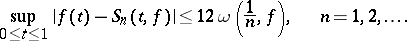The Haar system is a basis in the space,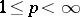. Ifand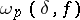is the integral modulus of continuity ofin the metric of, then (see )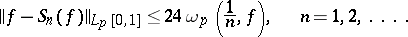The Haar system is an unconditional basis infor(see ).

Ifis Lebesgue integrable on, then its Fourier–Haar series converges to it at any of its Lebesgue points; in particular, almost-everywhere on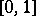. Here convergence (and absolute convergence) of the Fourier–Haar series at a fixed point ofdepends only on the values of the function in any arbitrarily small neighbourhood of this point.

For Fourier–Haar series the following properties differ substantially from each other: a) absolute convergence everywhere; b) absolute convergence almost-everywhere; c) absolute convergence on a set of positive measure; and d) absolute convergence of the series of Fourier coefficients. For trigonometric series all these properties are equivalent.

The properties of the Fourier–Haar coefficients differ sharply from those of the trigonometric Fourier coefficients. For example, if a functionis continuous on the intervaland if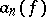are its Fourier coefficients with respect to the system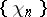, then the following inequality holds: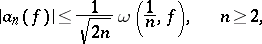which implies thatHowever, the Fourier–Haar coefficients of continuous functions cannot decrease too rapidly: Ifis continuous onand ifthen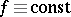on(see ).

For functions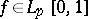,, the following estimates hold (see ):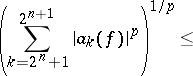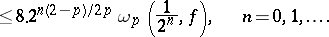Ifis of bounded variationon, then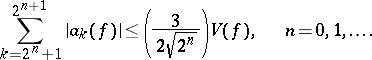All these inequalities are sharp in the sense of the order of decrease of their right-hand sides as(in the corresponding classes) (see ).

Almost-everywhere unconditionally-converging series of the form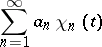(*)

are distinguished by an interesting peculiarity: If a series of the form (*) for any order of its terms converges almost-everywhere on a set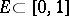of positive Lebesgue measure (the exceptional set of measure 0 may depend on the order of the terms of the series (*)), then this series converges absolutely almost-everywhere on. For series of the form (*) the following criterion holds: For a series (*) to converge almost-everywhere on a measurable setit is necessary and sufficient that the seriesconverges almost-everywhere on(see ).

Haar series may serve as representations of measurable functions: For any measurable functionthat is finite almost-everywhere onthere exists a series of the form (*) that converges almost-everywhere onto. Here the finiteness of the functionis essential: There is no series of the form (*) that converges to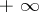(or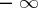) on a set of positive Lebesgue measure.

How to Cite This Entry:
Haar system. Encyclopedia of Mathematics. URL: http://encyclopediaofmath.org/index.php?title=Haar_system&oldid=40181
This article was adapted from an original article by B.I. Golubov (originator), which appeared in Encyclopedia of Mathematics - ISBN 1402006098. See original article